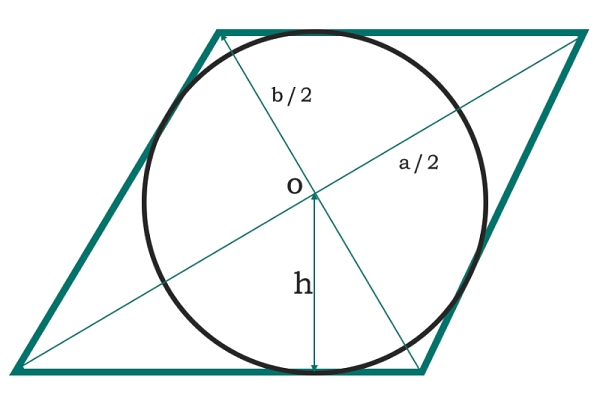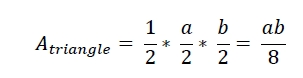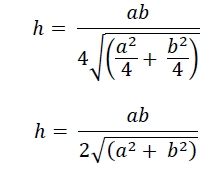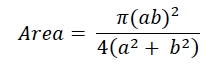# Area of circle inscribed within rhombus in C Program?

Here we will see the area of circle which is inscribed in a rhombus. The diagonals of the rhombus are ‘a’ and ‘b’. The radius of the circle is h.Two diagonals are creating four equal triangles. Each triangle is right-angled triangle, so their area is −Each side of the rhombus is the hypotenuses −So the area of the circle is −## Example

#include <iostream>
#include <cmath>
using namespace std;
float area(float a, float b) {
if (a < 0 || b < 0) //if the values are negative it is invalid
return -1;
float area = (3.1415 * (a*b * a*b))/(4 * (a*a + b*b));
return area;
}
int main() {
float a = 8, b= 10;
cout << "Area is: " << area(a, b);
}

## Output

Area is: 30.6488